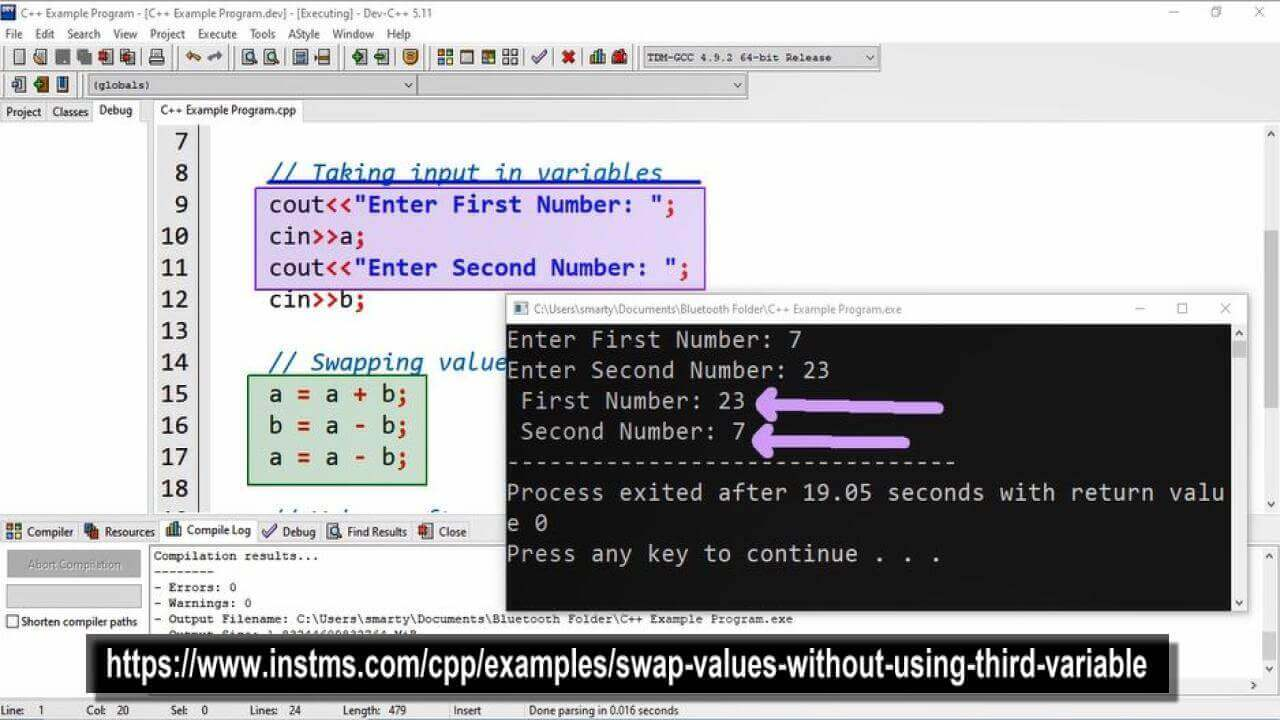# Swap Values Without Using Third Variable Program using CPP

## Source Code

#include <iostream>

using namespace std;

int main(int argc, char** argv) {

// Variable Declaration for input
int a,b;

// Taking input in variables
cout<<"Enter First Number: ";
cin>>a;
cout<<"Enter Second Number: ";
cin>>b;

// Swapping values
a = a + b;
b = a - b;
a = a - b;

// Values after swapping
cout<<" First Number: "<<a;
cout<<"\n Second Number: "<<b;

return 0;
}

## Output## Working

First of all two variables a and b are declared of type int. After this we take input in these variables using cin statement. Then swap these values using the following procedure.

• Get sum of both variables and assign it to first variable says a.
• Then deduct (subtract) value of variable b from total value (containing a).
• This deduction will result actual value of a (because total contains sum of both a and b).
• Now again we will deduct b (which now contains value of a after first deduction) from a.
• This will result in interchange value of these two variables without using third variable.